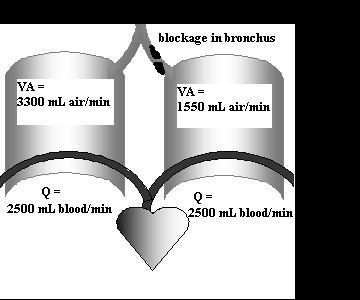Calculate ventilation/perfusion ratios

Ventilation

If you were helping someone measure their respiration, how would you explain the following terms to them?

Tidal Volume

Respiratory Rate

Minute Ventilation

If you measured their Tidal Volume and Respiratory Rate and got

TV = 500 mL/ breath and RR = 14 breaths/minute, what would their Minute Ventilation be in mL/minute?

Alveolar Ventilation is the amount of that air that actually gets down to the alveoli. If it takes 150 mL of air just to fill this person's trachea and bronchioles (their anatomical dead space), how much air gets into their alveoli on one 500 mL breath?How much air gets into their alveoli in one minute? This is alveolar ventilation or VA.

Application:

If this person were breathing through a snorkel two cm across and 20 cm long, how would it affect their alveolar ventilation? What if the snorkel were 2 meters long? (Hint – draw a diagram like the one above to set up this question. You can find the equation for volume of a tube on page 15).

Perfusion (Q) is how much blood the heart sends through the lungs per minute. It has to be just the same as the amount that goes through the body each minute. So perfusion for the two lungs, put together, is the same as cardiac output.

The lungs work best if they get both adequate air flow and adequate blood flow. This is expressed by the Ventilation: Perfusion ratio (VA/Q).  Its units are mL air/mL blood.

For the person in the first question, if perfusion is 5000 mL/minute, what is the ventilation: perfusion ratio for the lungs?

In a normal lung, the Ventilation: Perfusion ratio has a mean of 0.85. Here's a diagram of two lungs and the blood flow through them. Calculate the Ventilation: Perfusion ratio for each lung.If the ratio is too low, it means that the lung is getting enough blood but not enough air. The blood going through this lung isn't able to pick up Oxygen. This is wasted blood flow, because the heart had to work to pump it but the body doesn't get any Oxygen from it.

If the ratio is too high, the lung is

getting enough air but not enough blood. Most of the Oxygen inhaled is going back out, because there isn't enough blood going through the lung to pick it up. This is wasted ventilation; the body has to work to inhale, but doesn't benefit from the air inhaled. It is also called physiological dead space.

If you were in charge of blood flow to these two lungs, how would you adjust it?

You can demonstrate your understanding of these calculations by turning in a problem from the web page respiratory volumes quant problem. This problem must be done independently as specified in the contract you signed.